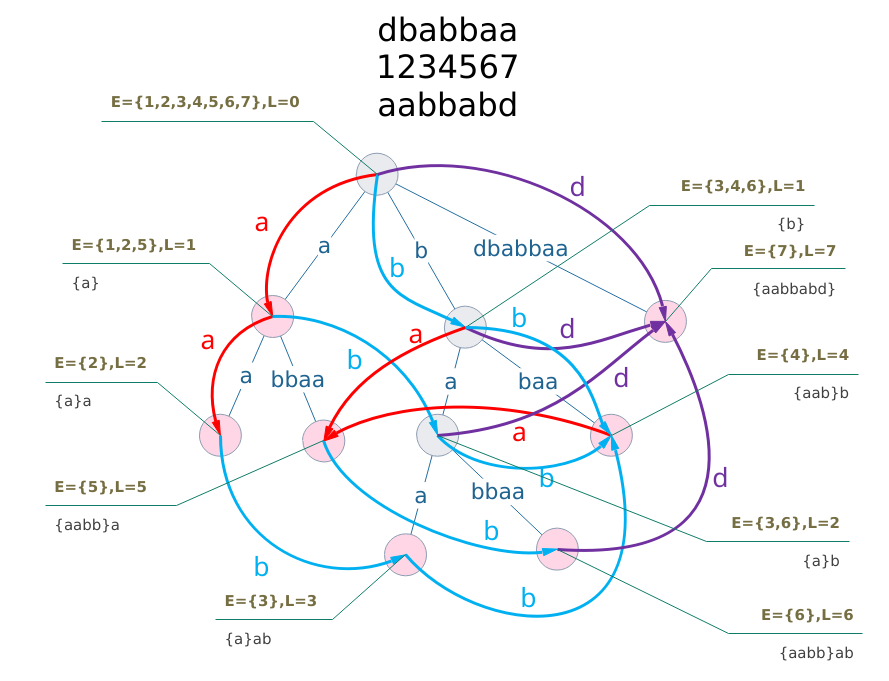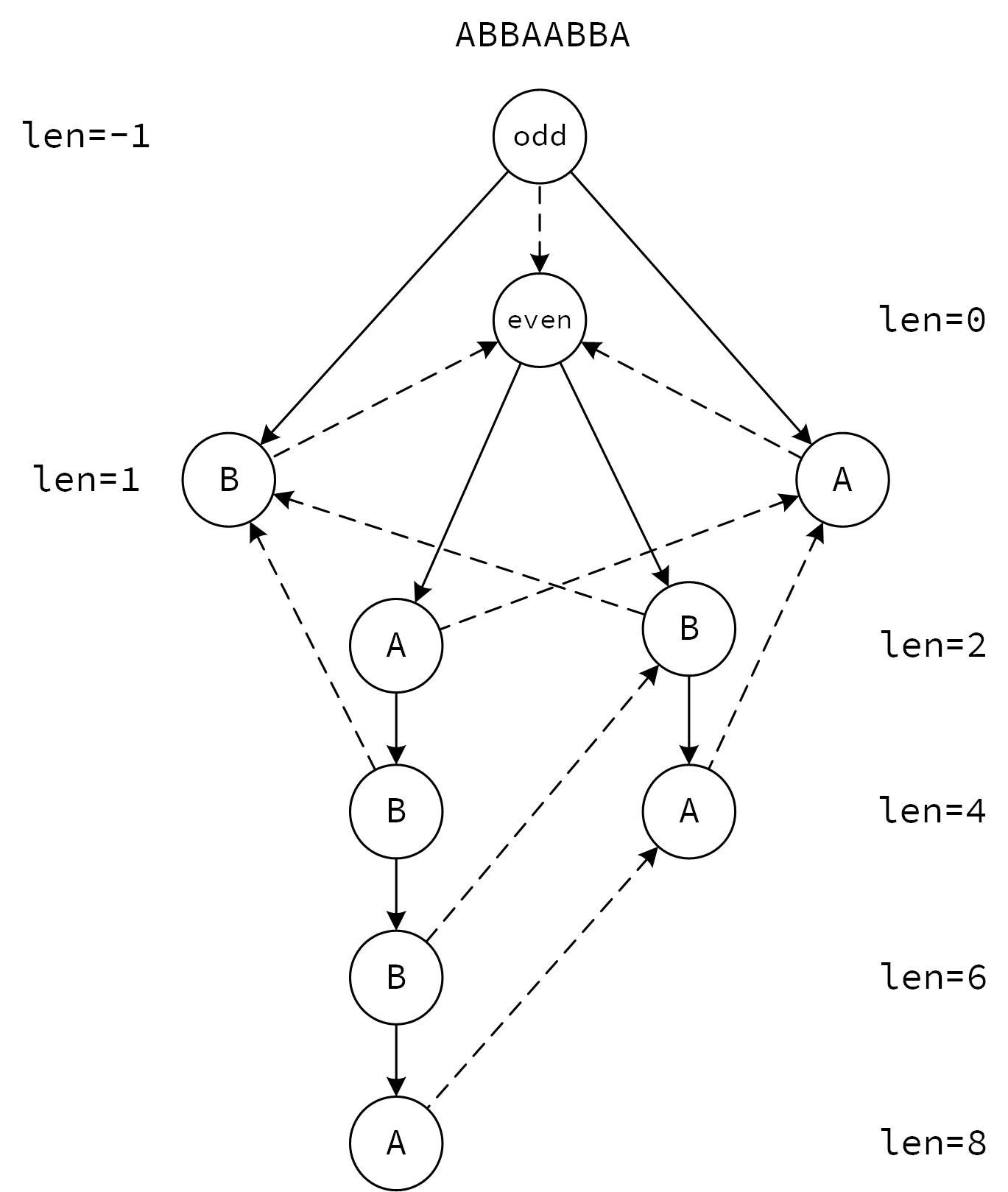## 后缀自动机

### 主要学习资料

• http://wyfcyx.is-programmer.com/posts/76107.html （对我的帮助最大）
• http://www.cnblogs.com/Robert-Yuan/p/5187439.html （相当详细）
• 2012年noi冬令营陈立杰讲稿.ppt （始祖）
• hiho一下 127周128周。（理论结合实践）
• https://chrt.github.io/2017/04/21/suffix-automaton/ （看到这个我觉得我不用再写这篇文章了）
• https://zhuanlan.zhihu.com/p/25948077 （复杂度“证明”）

• SAM 是一种确定有限状态自动机 (DFA)，它能够接受所有字符串的所有后缀。
• 重要的信息有 $$tranc(x, c)$$$$fa(x)$$，有两种理解方式：
• 自动机形态
• $$tranc(x,c)$$ 为状态 $$x$$ 接受字符 $$c$$ 后到达的状态
• $$fa(x)$$$$right(x)$$ 的超集中最小的所对应的状态
• 后缀树形态（逆序）
• $$fa(x)$$$$x$$ 的父节点
• $$tranc(x,c)$$$$x$$ 对应的后缀在首部增加字符 $$c$$ 后所形成的后缀在树中对应的结点
• 无论那种理解方式，反正可以互相转换。鉴于后缀树更为直观、简洁，那么就以这种方式来描述。
• 对于一个字符串 $$s(|s|=n)$$，建立它的后缀 trie，就形成了它的后缀树，但这样效率和空间都很糟糕。如果对 trie 中的单链进行压缩，那么可以证明，结点个数是 $$O(n)$$ 的。（证明：借用虚树复杂的证明，$$n$$ 个叶节点都是关键点，而关键点两两之间的 LCA 不超过 $$n-1$$，所以总的结点数不超过 $$2n-1$$ 个。）
• 接下来的关键是如何在线性时间内构造一棵压缩后的后缀树。
• 先给张图，未完待续。### 一些性质

• $$fa$$ 指针组成的是反串的后缀树，当然是树了。$$tranc$$ 指针组成的是 DFA，所以是一个有向无环图（DAG），存在拓扑序。

• $$tranc$$ 指针使 $$len$$ 增加，$$fa$$ 指针使 $$len$$ 减少。
• 关键点（红色标记）自带一个 $$EndPos$$，然后每个结点的 $$EndPos$$ 集合是自己和子节点的 $$EndPos$$ 集合的并集，且子节点以及本身的 $$EndPos$$ 集合之间没有交集。
• 接受状态是 $$last$$ 状态到根的链上的所有状态。
• $$len$$ 的大小是父节点的 $$len$$ 加上父边压缩的字符数。
• $$[len_{min},len_{max}]$$ 的区间大小是由 $$tranc$$ 转移过来的状态的区间大小之和。

### 一些套路

• 如果要快速找到一个子串对应的状态，可以在后缀树上倍增。比如要找 [l, r] 的子串，那么找到 [1, r] 逆序的所在的关键点，然后往上爬 l - 1 个长度。
• $$cnt$$ 表示该状态的 $$EndPos$$ 集合的大小，可以在建完自动机后累加得到。
• 如果要进行多串匹配的话，不妨使用广义后缀自动机，往往会简单一些。
• 如果可以离线，可以用树上启发式合并得到每个点的 EndPos 集合。
• 如果要维护 EndPos 相关的信息，来一发 LCT。
• 获取拓扑序可以按照 $$len$$ 进行基数排序。
• 为了某个状态具体的字符串内容，可以记录 $$EndPos$$ 中的某一个元素。

## 回文自动机• 一个结点只表示一个回文串，这个特性会带来极大的方便。
• 对于 $$tranc$$ 边来说，奇数和偶数都是树的形式。
• 多了一个 $$num$$ 表示状态对应的回文串的后缀中回文串的个数（以 $$fa$$ 为边的树的深度）。
• $$tranc$$ 边很可能比 $$fa$$ 边有用。

• $$fa$$ 指针使 $$len$$ 减少。
• $$cnt$$ 的含义和计算方法相同。